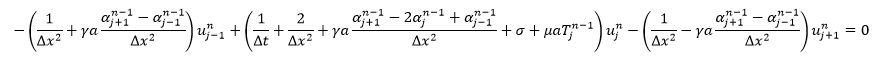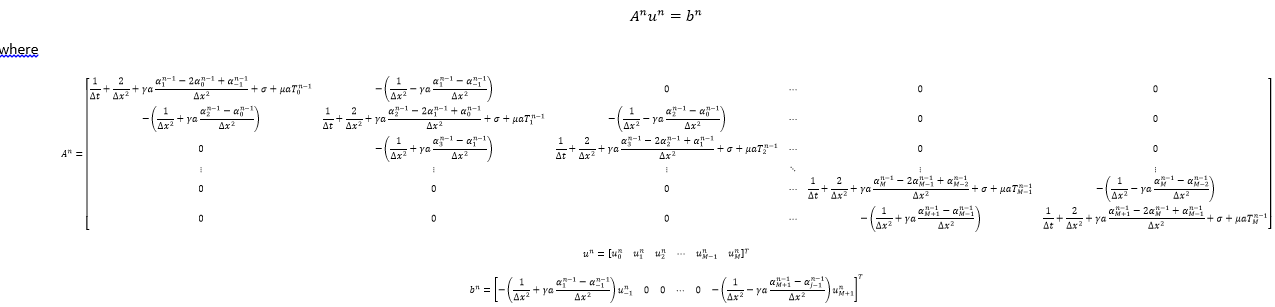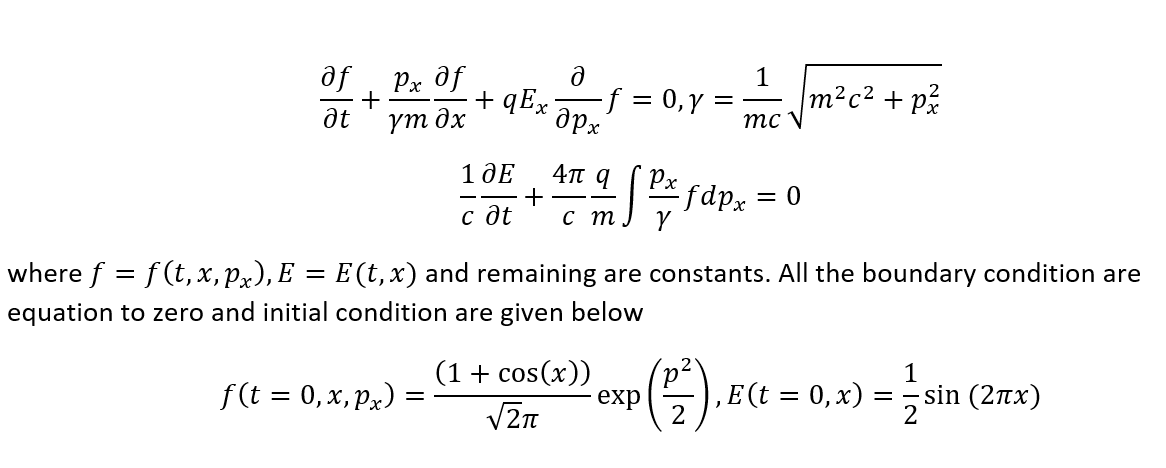## System of equation into matrix for each "n"...

Dear Users!

I hope you are doing well. I have the following discretized formfor n>=1 and j=0..M. We obtained the following matrix equation for any "n" and j=0..M as:I want matrix proc of any useful way to define A^n, u^n, and b^n. I am waiting for your positive response. Thanks in advancs

## Why are the level curves wrong when axis mode is ...

Using plot3d(..., style=surfacecontour, ...) or contourplot3d(...) displays wrong level curves when some axis are switched to a log mode.

Example:

 > restart:
 > interface(version)(1)
 > X := (0.4000000000e-4*(-R+80.00))/(R*(0.4e-1+M__a)):
 > plot3d(X, R=0..10, M__a=10^0..10^4, style=surfacecontour, color=gold)> plot3d(X, R=0..10, M__a=10^0..10^4, axis=[mode=log], axis=[mode=log], style=surfacecontour, color=gold)> plots:-display(   plots:-contourplot3d(X, R=0..10, M__a=10^0..10^4, axis=[mode=log], axis=[mode=log], color=red),   plot3d(X, R=0..10, M__a=10^0..10^4, style=surface, color=gold) )>

The problem is not dramatic because there is a workaround.

 > restart:
 > interface(version)(1)
 > X := (0.4000000000e-4*(-R+80.00))/(R*(0.4e-1+M__a)):
 > fig := plot3d(X, R=0..10, M__a=10^0..10^4, style=surfacecontour, color=gold): Tr  := plottools:-transform((x, y, z) -> [x, log(y), log(z)]): plots:-display(Tr(fig), axis=[tickmarks=[seq(i=10^i, i=0..4)]], axis=[tickmarks=[seq(i=nprintf("%1.0e", 10.^i), i=-7..-1)]])>

## Why does dsolve return a solution with a singular...

I don't understand why the solution of sys_2 isn't those of sys_1 when M__p=1 and M__a=0 ?

Traces of the computation seem to indicate that dsolve proceeds exactly the same for sys_2 and sys_1 .

Please note that sol_1 contains a term of the form t*cos(t) that sol_2 doesn't, thus the question: "Is sol_2 correct?"

Could you help me to fix this?
TIA

 > restart
 > infolevel[dsolve] := 4;(1)
 > sys_1 := {diff(x(t), t\$2)=sin(t)-x(t), x(0)=0, D(x)(0)=0}; sol_1 := dsolve(sys_1)Methods for second order ODEs: --- Trying classification methods --- trying a quadrature trying high order exact linear fully integrable trying differential order: 2; linear nonhomogeneous with symmetry [0,1] trying a double symmetry of the form [xi=0, eta=F(x)] -> Try solving first the homogeneous part of the ODE    checking if the LODE has constant coefficients    <- constant coefficients successful    -> Determining now a particular solution to the non-homogeneous ODE       building a particular solution using variation of parameters <- solving first the homogeneous part of the ODE successful(2)
 > sys_2 := {(M__p+M__a)*diff(x(t), t\$2)=M__p*sin(t)-x(t), x(0)=0, D(x)(0)=0}; sol_2 := dsolve(sys_2)Methods for second order ODEs: --- Trying classification methods --- trying a quadrature trying high order exact linear fully integrable trying differential order: 2; linear nonhomogeneous with symmetry [0,1] trying a double symmetry of the form [xi=0, eta=F(x)] -> Try solving first the homogeneous part of the ODE    checking if the LODE has constant coefficients    <- constant coefficients successful    -> Determining now a particular solution to the non-homogeneous ODE       building a particular solution using variation of parameters <- solving first the homogeneous part of the ODE successful(3)
 > eval(sol_2, [M__p=1, M__a=0])
 >

PS: Already, in the following case, dsolve doesn't return the solution of sys_1.

```sys_3 := {(A+B)*diff(x(t), t\$2)=(A+B)*sin(t)-x(t), x(0)=0, D(x)(0)=0};
sol_3 := dsolve(sys_3)
```

If I do this

```sys_4 := {(A+B)*diff(v(t), t)=(A+B)*sin(t)-x(t), diff(x(t), t)=v(t), x(0)=0, v(0)=0}:
sol_4 := dsolve(sys_4)
```

I get a very complex solution wich contains a piecewise function which separates the cases A+B=1 and A+B<>1.
Evaluating sol_4 for A+B=1 gives the same expression than sys_1:

```simplify(eval(sol_4, A=1-B), trig)
/       1                  1          1         \
{ v(t) = - sin(t) t, x(t) = - sin(t) - - cos(t) t }
\       2                  2          2         /
```

Here is a workaround to get the correct solution of sys_2:

```sys_5 := {(M__P+M__A)*diff(v(t), t)=(M__P+C)*sin(t)-x(t), diff(x(t), t)=v(t), x(0)=0, v(0)=0}:
sol_5 := dsolve(sys_5):
simplify(eval(sol_5, [M__P=1, M__A=0, C=0]), trig)
/       1                  1          1         \
{ v(t) = - sin(t) t, x(t) = - sin(t) - - cos(t) t }
\       2                  2          2         /
```

e

## How to setup pacemaker and Corosync to maple work?...

Can pacemaker and corosync work with maple?

How to setup pacemaker and Corosync to maple work in Amazon EC2?

how to make it work in clusters ?

what is the difference with supercomputing in Amazon ?

which consulting company in Hong Kong can help to use supercomputing in Amazon in my case ? I would like to run batch of batch total 100 script running maple in one instance , but total numbers need to run around 60 years. Any consultant to calculate and setup this supercomputing or pacemaker to make calculations into one day or a few days ?

## How can i see maple calculations?...

How can i see analytical maple calculations?

## Solve command Issue due to Computational Cost...

Hello Everyone;

Hope you are fine. I am solving system of odes using rk-4 method. For this purpose I formulate the "residual" (on maple file) which is further function of "x" and "y". With the help of discritization point further I convert "residual" into system of ode's. Then i used "sys111 := solve(odes_Combine, `~`[diff](var, t))" to simplify the system. Finnally i applied RK-1. Code is pasted and attached. This all process is for "N=4". When i increase the value of "N", number of Odes increase accordingly. With increasing value of "N" the comand "sys111 := solve(odes_Combine, `~`[diff](var, t))" taking a lot of time due to heavy computation. Is that any way to proceed without this comand for rk-1?

Question1.mw

 >(1)
 >>(2)
 >>>>(3)
 >>>>>>## Numerical Scheme for Integro-differential equation...

Hello;

Hope you are fine. Can i apply numerical scheme on maple for the following problem. This in integro-differential equation i think. Waiting for kind response.

Thanks## How to declare variables local with assign?...

I need to declare a whole set of variables as local. The variable names are generates algorithmically using assign. Like so:

`seq(seq(assign(cat(S,i,j)=Vector(datatype=float)),i=1..9),j=1..9);`

Stand-alone, this works and creates all these Vectors for later use. But this:

`local seq(seq(assign(cat(S,i,j)=Vector(datatype=float)),i=1..9),j=1..9);`

does not work; I get an "error; '(' unexpected".

I really do not want to type all these by hand... on the other hand, if I do not declare these as local I get 99 warnings about implicit local declaration; not nice.

Is there a way to do this?

Thanks,

M.D.

PS: I do not upload as the one line really is all that is needed. At the lowest level one does not get the implicit-declaration warning, but with "local" it still fails.

## Problem in comparing the coefficient of exponentia...

Dear Useres!

Hope everyone is fine here! I want to compare the coeficient of exp(k*eta+m*eta+n*eta) for k=0,1,2,3,...,N,n=0,1,2,3,...,N and m=0,1,2,3,...,N for N=10 in the following attached file. But I got some error, please have a look and try to fix it as early as possible. Please take care and thanks

Compare_coeff.mw

## How to find the maximum value of this function?...

Hi,

I solve numerically an ODE system which depends on 25 parameters.
I want to know the maximum value of the time at which a specific event is triggered, when these parameters vary (independently the one of the other) within a 25 dimensional hyperbox.

To solve this maximization problem, which I assume is local1, I would like to use NLPSolve

The attached zip (I use Mac OSX) contains:

• a file that gives a full description of the problem (the original ".pages" file and its export as pdf),
• an m file wich contains the solution procedure plus a few other variables needed to solve the problem,
• the mw file which contains:
• the reading of the m file,
• the procedure (OBJ) which returns the trigerring time,
• many attemps to find its maximum value using NLPSolve.

None of my attempts at using NLPSolve gave me the expected answer

Could you help me to fix this?

mw_and_m_files.zip

1: Initializing a local method (see the explanation file) with different points gave me different optimizers but all of them led to rather close values of the objective function. Thus the problem is either global instead of local, or convergence might not be achieved for all the initialization points (but keep in mind that I'm not interested in the location of the minimizer(s) but in the maximum value of if the time when the event is triggered).

## Problem in displaying the edge weights of some dir...

It seems that DrawGraph cannot display the weights of more than 45 arcs of a directed graphs (the arcs themselves are keeping to be displayed correctly).

Maybe a version issue?

WeightedGraphs.mw

## How to pkill or kill mserver when using Grid?...

I rewrite my code with Grid library using local vCPU of amazon

discover no license of distributed HPC when setup command show need go to acresso.com

can Grid still be used and function with local 96 number of vCPU?

then when I test code, I can not pkill mserver in ps -aux in LInux

can the performance really improved ? Because I suppose 3 to 5 minutes mserver will end and disappear from ps -aux but grid node of mserver still running

originally I can run 100 batches every day., but I have to monitor decrease of memory in order to determine whether can kill mserver for next batch

I expect run 400 batches per day. But Can not kill mserver when using grid

In my code I had using time limit(30, ...)

when using Grid seq of function , can lprint work normally to get results into text ?

i notice Grid up to 57, do I need to recalculate and revise code to fit 96 vCPU for grid number 96?

## Simplify an expression in special way...

Dear Users!

Hope everything going find with you. I want to generate sequence of Spline[i,p], p=1…M as given bellow

restart; M := 3;
for p while p <= M do
(x-x[i])*B[i, p-1]/(x[i+p]-x[i])+(x[i+p+1]-x)*B[i+1, p-1]/(x[i+p+1]-x[i+1]);
B[i, p] := collect(%, [`\$`(B[i+s, 0], s = 0 .. M)]);
B[i+1, p] := eval(%, i = i+1)
end do;
for p while p <= M do
Spline[i, p] := B[i, p]
end do

The expression for Spline[i,1] and Spline[i,2] are fine but the expressions of Spline [i,p] p>2 is not in simplified form I got the its simplification like given bellow for Spline[i,3]

Spline[i, 3]:=(x-x[i])^3*B[i, 0]/((x[i+3]-x[i])*(x[i+2]-x[i])*(x[i+1]-x[i]))+(((x-x[i])*(x-x[i]))*(x[i+2]-x)/((x[i+3]-x[i])*(x[i+2]-x[i])*(x[i+2]-x[i+1]))+(x-x[i])*(x[i+3]-x)*(x-x[i+1])/((x[i+3]-x[i])*(x[i+3]-x[i+1])*(x[i+2]-x[i+1]))+(x[i+4]-x)*(x-x[i+1])^2/((x[i+4]-x[i+1])*(x[i+3]-x[i+1])*(x[i+2]-x[i+1])))*B[i+1, 0]+((x-x[i])*(x[i+3]-x)^2/((x[i+3]-x[i])*(x[i+3]-x[i+1])*(x[i+3]-x[i+2]))+(x[i+4]-x)*(x-x[i+1])*(x[i+3]-x)/((x[i+4]-x[i+1])*(x[i+3]-x[i+1])*(x[i+3]-x[i+2]))+((x[i+4]-x)*(x[i+4]-x))*(x-x[i+2])/((x[i+4]-x[i+1])*(x[i+4]-x[i+2])*(x[i+3]-x[i+2])))*B[i+2, 0]+(x[i+4]-x)^3*B[i+3, 0]/((x[i+4]-x[i+1])*(x[i+4]-x[i+2])*(x[i+4]-x[i+3]));

I am waiting for positive respone. Please take care and thanks in advance

## partial differential equations, PDEs...

Hi,

I want to solve this system of PDEs. Please let me know how I can solve it.

Kindly find both my code written in Maple and the image of the problem.

Best,PDE.mw

## Need to reduce the Jacobian matrix construction as...

Dear Users!
Hope everyone fine here. I tried (as given bellow) to find the solution of nonlinear system of PDEs via FDM. To solve system of nonlinear equations I used newton raphson method and for higher value of like Mx > 8 the matrix G and G1 (mentioned as red) take alot of time. Can anyone help me to reduce the computational time? Becuase I have to evealuate the solution for Mx = 50.

restart; Digits := 30; with(LinearAlgebra);
T := 1; L := 3; N := 30; Mx := 5; `&Delta;x` := L/(1.*Mx); `&Delta;t` := T/(1.*N);
for i from 0 while i <= Mx do
u[i, 0] := 0.; u[i, -1] := u[i, 1]; tau[i, 0] := 0.; theta[i, 0] := 0.; theta[i, -1] := theta[i, 1]
end do;
for n from 0 while n <= N do u[0, n] := 0.; u[Mx, n] := 0.; theta[0, n] := 1.; theta[Mx, n] := 0.
end do;
for n from 0 while n <= N-1 do
print("Simulation in proccess at time-level n", n+1);
for i while i <= Mx-1 do
Ru[i, n] := simplify((u[i+1, n+1]-u[i+1, n])/`&Delta;t`+(u[i+1, n+1]-2*u[i+1, n]+u[i+1, n-1])/`&Delta;t`^2-(u[i+1, n+1]-2*u[i, n+1]+u[i-1, n+1])/`&Delta;x`^2+25.*(u[i+1, n+1]+(u[i+1, n+1]-u[i+1, n])/`&Delta;t`)-1.5*(theta[i, n]+(theta[i, n+1]-theta[i, n])/`&Delta;t`));
`R&theta;`[i, n] := simplify((theta[i+1, n+1]-theta[i+1, n])/`&Delta;t`+(theta[i+1, n+1]-2*theta[i+1, n]+theta[i+1, n-1])/`&Delta;t`^2-(theta[i+1, n+1]-2*theta[i, n+1]+theta[i-1, n+1])/((15.)*`&Delta;x`^2)-((u[i, n+1]-u[i-1, n+1])/`&Delta;x`)^2/(3.)) end do;
for i while i <= Mx-1 do
`R&tau;`[i, n] := simplify(tau[i+1, n+1]+(tau[i+1, n+1]-tau[i+1, n])/`&Delta;t`-1.5^(-1/4)*(u[i+1, n+1]-u[i, n+1])/`&Delta;x`)
end do;
Sys := `<,>`(seq(Ru[i, n], i = 1 .. Mx-1), seq(`R&tau;`[i, n], i = 1 .. Mx-1), seq(`R&theta;`[i, n], i = 1 .. Mx-1));
V := `<,>`(seq(u[i, n+1], i = 1 .. Mx-1), seq(theta[i, n+1], i = 1 .. Mx-1), seq(tau[i, n+1], i = 2 .. Mx));
G := Matrix(3*(Mx-1), proc (i, j) options operator, arrow; diff(Sys[i], V[j]) end proc); G1 := MatrixInverse(G);
X[n, 0] := Vector(1 .. 3*(Mx-1), 1);
for k1 from 0 to r do
X[n, k1+1] := eval(V-G1 . Sys, Equate(V, X[n, k1]))
end do;
Sol[n] := Equate(V, X[n, r+1]); assign(op(Sol[n]));
if n > 0 then
U := eval(`<,>`(seq(u[i1, n+1], i1 = 1 .. Mx)-seq(u[i1, n], i1 = 1 .. Mx))); Noru[n+1] := Norm(%, 2); print("L[&infin;] norm of &tau;(x,y,t) at time level = ", %);
Theta := eval(`<,>`(seq(theta[i1, n+1], i1 = 0 .. Mx)-seq(theta[i1, n], i1 = 0 .. Mx))); `Nor&theta;`[n+1] := Norm(%, 2); print("L[&infin;] norm of &theta;(x,y,t) at time level = ", %) else print("n < 0")
end if end do

Special request to:
@acer @Carl Love @Kitonum @Preben Alsholm

 1 2 3 4 5 6 7Last Page 1 of 71
﻿